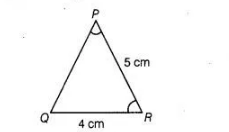# In ΔPQR, if ∠R = ∠P and QR = 4 cm`
Question:

In ΔPQR, if ∠R = ∠P and QR = 4 cm and PR = 5 cm. Then, the length of PQ is

(a) 4 cm

(b) 5 cm

(c) 2 cm

(d) 2.5 cm

Solution:

(a) Given, $\triangle P Q R$ such that $\angle R=\angle P, Q R=4 \mathrm{~cm}$ and $P R=5 \mathrm{~cm}$In $\triangle P Q R$, $\angle R=\angle P$

$\Rightarrow \quad P Q=Q R \quad$ [sides opposite to equal anqles are equal]

$\Rightarrow \quad P Q=4 \mathrm{~cm} \quad[\because Q R=4 \mathrm{~cm}]$

Hence, the length of $P Q$ is $4 \mathrm{~cm}$.